Updated date:

# Gamma Ray Spectroscopy

Dr. Thomas Swan is a published physicist who received his Ph.D. in nuclear astrophysics from the University of Surrey.

## What is Gamma Ray Spectroscopy?

If you recognize that dog whistles emit ultrasonic sound that is inaudible to the human ear, then you can understand gamma rays as a form of light that is invisible to the human eye. Gamma rays are an ultra high frequency of light that is emitted by radioactive elements, energetic celestial bodies such as black holes and neutron stars, and high energy events such as nuclear explosions and supernovae (the death of stars). They are referred to as radiation because they can penetrate deep into the human body, causing harm when their energy is deposited.

In order to use gamma rays safely, the source and energy of their emission must be determined. The invention of gamma ray detectors allowed this function to be performed by identifying dangerous gamma-emitting elements. Recently, detectors placed aboard space telescopes have allowed humanity to determine the composition of other planets and stars by measuring their gamma emissions. These types of studies are collectively referred to as gamma ray spectroscopy.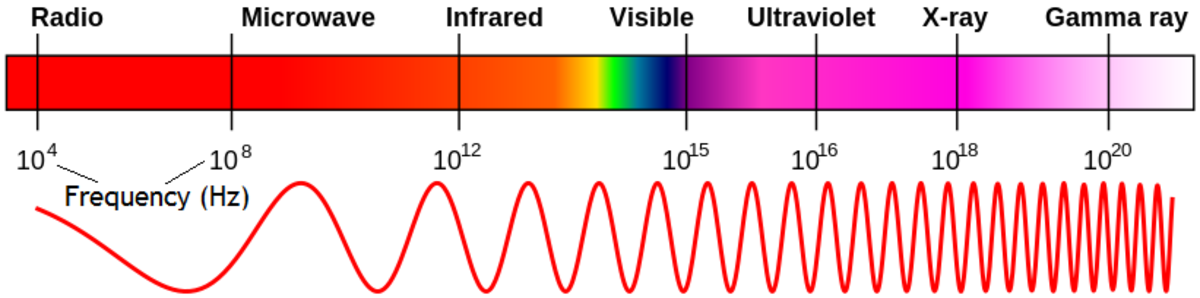Gamma rays are the highest frequency of light. There is only a small region of the electromagnetic (light) spectrum that is visible to the human eye.Inductiveload, NASA, via Wikimedia CommonsElectrons circle the nucleus of the atom in orbits.Picasa Web Albums (Creative Commons)

## Gamma Ray Detectors

Gamma ray detectors are made from semiconductor materials, which contain atoms with orbiting electrons that can easily absorb the energy of a passing gamma ray. This absorption pushes the electron into a higher orbit, allowing it to be swept away in an electrical current. The lower orbit is called the valence band, and the higher orbit is called the conduction band. These bands are close together in semiconductor materials such that valence electrons can easily join the conduction band by absorbing the energy of a gamma ray. In germanium atoms, the band-gap is only 0.74 eV (electron volts), making it an ideal semiconductor for use in gamma ray detectors. The small band-gap means only a small amount of energy is required to produce a charge carrier, resulting in large output signals and high energy resolution.

To sweep the electrons away, a voltage is applied to the semiconductor to create an electric field. To help achieve this, it is infused, or doped, with an element that has fewer valence band electrons. These are called n-type elements, having only three valence electrons compared with the semiconductor’s four. The n-type element (e.g. lithium) drags electrons away from the semiconductor material, becoming negatively charged. By applying a reverse biased voltage to the material, this charge can be pulled towards a positive electrode. The removal of electrons from the semiconductor atoms creates positively charged holes that can be pulled towards a negative electrode. This depletes charge carriers from the center of the material, and by increasing the voltage, the depletion region can be grown to encompass most of the material. An interacting gamma ray will create electron-hole pairs in the depletion region, which are swept up in the electric field and deposited on the electrodes. The collected charge is amplified and converted to a voltage pulse of a measurable size that is proportional to the energy of the gamma ray.

As gamma rays are an extremely penetrating form of radiation, they require large depletion depths. This can be achieved by using large germanium crystals with impurities of less than 1 part in 1012 (a trillion). The small band-gap requires the detector to be cooled to prevent noise from leakage current. Germanium detectors are therefore placed in thermal contact with liquid nitrogen with the whole setup housed within a vacuum chamber.

Europium (Eu) is a metallic element that commonly emits gamma rays when it has a mass of 152 atomic units (see nuclear chart). Below is a gamma ray spectrum that was observed by placing a small lump of 152Eu in front of a germanium detector.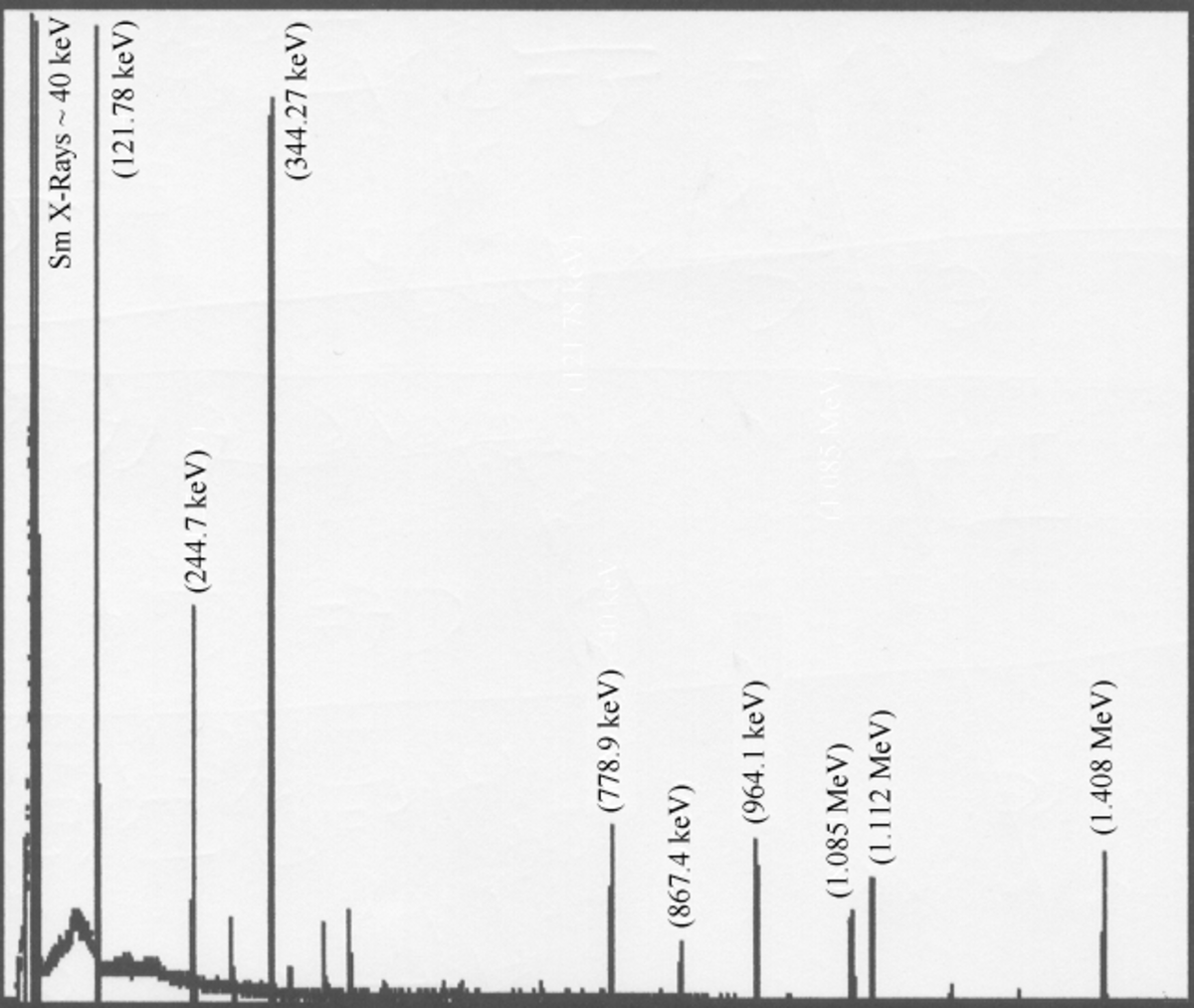Europium-152 gamma ray spectrum. The larger the peak, the more frequent the emission from the europium source. The energies of the peaks are in electron volts.

## Energy Calibration of Germanium Gamma Ray Detectors

This article will now detail the typical processes employed in gamma ray spectroscopy. The above spectrum was used to calibrate the energy scale of a Multi-Channel Analyzer (MCA). 152Eu has a wide range of gamma ray peaks, allowing for a precise energy calibration up to around 1.5 MeV. Five of the peaks were tagged in the MCA with their previously determined, known energies, thus calibrating the energy scale of the equipment. This calibration allowed the energy of gamma rays from unknown sources to be measured to an average uncertainty of 0.1 keV.

## Background Spectrum

With all laboratory sources shielded from the detector, a spectrum was recorded to measure gamma rays emerging from the surrounding environment. This background data was allowed to accumulate for 10 minutes. A number of gamma ray peaks were resolved (below). There is a prominent peak at 1.46 MeV that is consistent with 40K (potassium). The most likely cause is the concrete that makes up the laboratory building. 40K makes up 0.012% of all naturally occurring potassium, which is a common constituent in building materials.

214Bi and 214Pb (bismuth and lead) are produced following the decay of uranium within the Earth, and 212Pb and 208Tl (lead and thallium) follow the decay of thorium. 137Cs (cesium) can be found in the air as a result of past nuclear weapons testing. The small 60Co peaks (cobalt), could be attributed to less than adequate shielding of the detector from this intense laboratory source.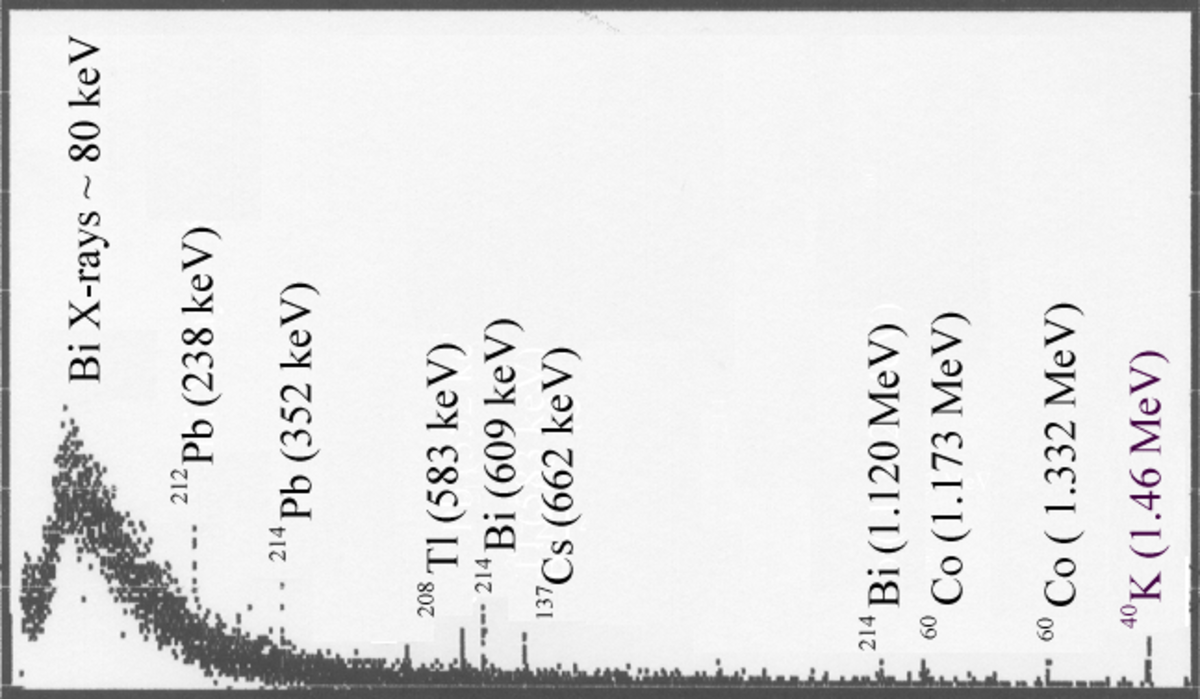The spectrum of background gamma rays within a normal concrete building.

## X-Rays in the Europium Spectrum

At around 40 keV, a number of x-rays were detected in the europium spectrum. X-rays have a lower energy than gamma rays. They are resolved below in a magnified image of this region of the spectrum. The two large peaks have energies of 39.73 keV and 45.26 keV, which correspond to the x-ray emission energies of 152Sm. Samarium is formed through the capture of an inner electron from 152Eu in the reaction: p+e → n+ν. X-rays are emitted as electrons descend to fill the vacancy of the captured electron. The two energies correspond to electrons that come from two different shells, known as the Kα and Kβ shells.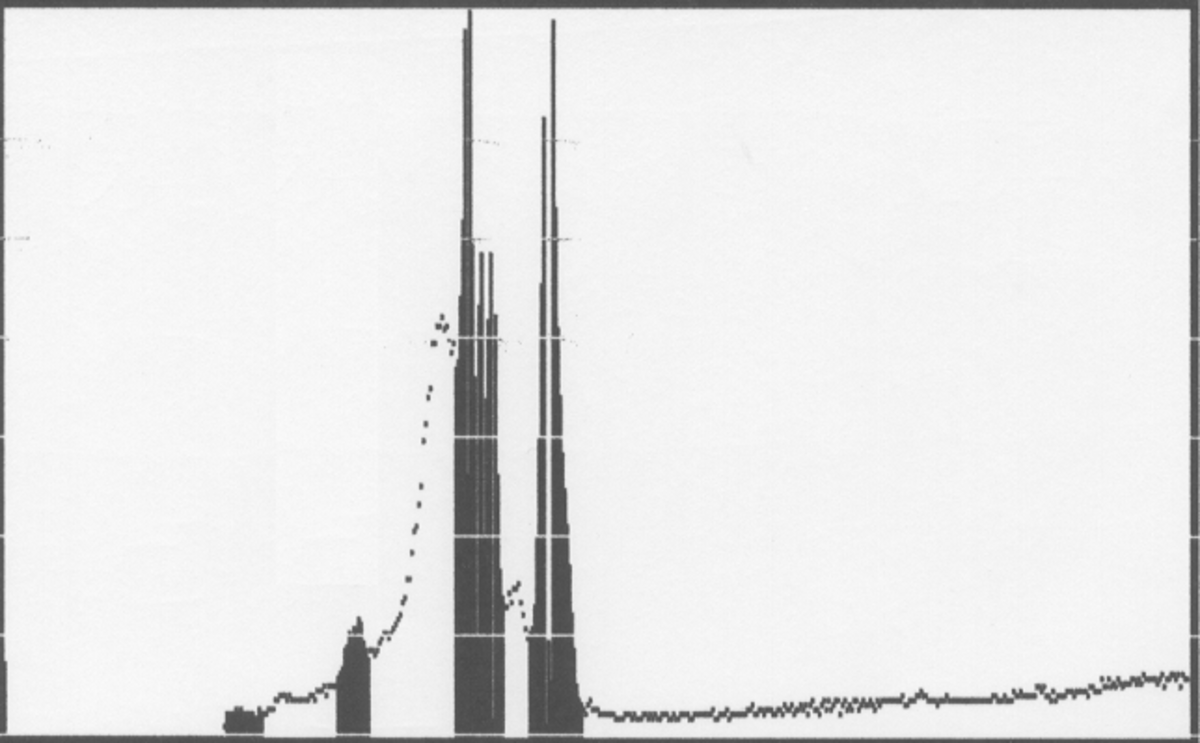Zooming in at the low energy end of the europium spectrum to see samarium x-rays.

## X-Ray Escape Peaks

The small peak at even lower energy (~30 keV) is evidence for an x-ray escape peak. X-rays are low energy, which increases the chance of them being photoelectrically absorbed by the germanium detector. This absorption results in a germanium electron being excited to a higher orbit, from which a second x-ray is emitted by the germanium to return it to it’s ground state electron configuration. The first x-ray (from samarium) will have a low penetration depth into the detector, increasing the chance that the second x-ray (from germanium) will escape from the detector without interacting at all. As the most intense germanium x-ray occurs at an energy of ~10 keV, the detector records a peak at 10 keV less than the samarium x-ray that was absorbed by the germanium. An x-ray escape peak is also evident in the spectrum of 57Co, which has many low-energy gamma rays. It can be seen (below) that only the lowest energy gamma ray has a visible escape peak.

## Peak Summing

A relatively high activity 137Cs source was placed close to the detector, producing a very large count rate, and yielding the spectrum below. The energies of a barium x-ray (32 keV) and a cesium gamma ray (662 keV) have occasionally summed to produce a peak at 694 keV. The same is true at 1324 keV for the summing of two cesium gamma rays. This occurs during a high count rate because the probability of a second ray penetrating the detector before the charge from the first ray is collected increases. As the amplifier shaping time is too long, the signals from the two rays are summed together. The minimum time that must separate two events is the pile-up resolution time. If the detected signal pulse is rectangular, and the two signals overlap, the result will be a perfect summing of the two signals. If the pulse is not rectangular, the peak will be poorly resolved, as in many cases the signals will not add at the full amplitude of the signal.

This is an example of random summing, as other than their coincidental detection, the two signals are unrelated. A second kind of summing is true summing, which occurs when there is a nuclear process dictating a quick succession of gamma ray emissions. This is often the case in gamma ray cascades, where a nuclear state with a long half life decays to a short-lived state that quickly emits a second ray.

## Annihilation Photons

22Na (sodium) decays by positron emission (β+) in the reaction: p → n+e++ν. The daughter nucleus is 22Ne (neon) and the state occupied (99.944% of the time) is a 1.275 MeV, 2+ nuclear state, which subsequently decays via gamma rays to the ground state, producing a peak at that energy. The emitted positron will annihilate with an electron within the source material to produce back-to-back annihilation photons with energies equal to the rest-mass of an electron (511 keV). However, a detected annihilation photon can be shifted down in energy by a few electron volts due to the binding energy of the electron involved in the annihilation.

The width of the annihilation peak is uncharacteristically large. This is because the positron and electron occasionally form a short-lived orbiting system, or exotic atom (similar to hydrogen), called positronium. The positronium has a finite momentum, meaning that after the two particles annihilate each other, one of the two annihilation photons may possess slightly more momentum than the other, with the sum still being twice the rest-mass of the electron. This Doppler effect increases the energy range, broadening the annihilation peak.

## Energy Resolution

The percentage energy resolution is calculated using: FWHM ⁄ Eγ (×100%), where Eγ is the gamma ray energy. The full width at half maximum (FWHM) of a gamma ray peak is the width (in keV) at half the height. For a 152Eu source at 15 cm from a germanium detector, the FWHM of seven peaks were measured (below). We can see that the FWHM increases linearly as the energy increases. Conversely, the energy resolution decreases. This occurs because high energy gamma rays produce a large number of charge carriers, leading to increased statistical fluctuations. A second contributor is incomplete charge collection, which increases with energy because more charge needs to be collected in the detector. Electronic noise provides a minimum, default peak width, but it is invariant with energy. Also note the increased FWHM of the annihilation photon peak due to the Doppler broadening effects described earlier.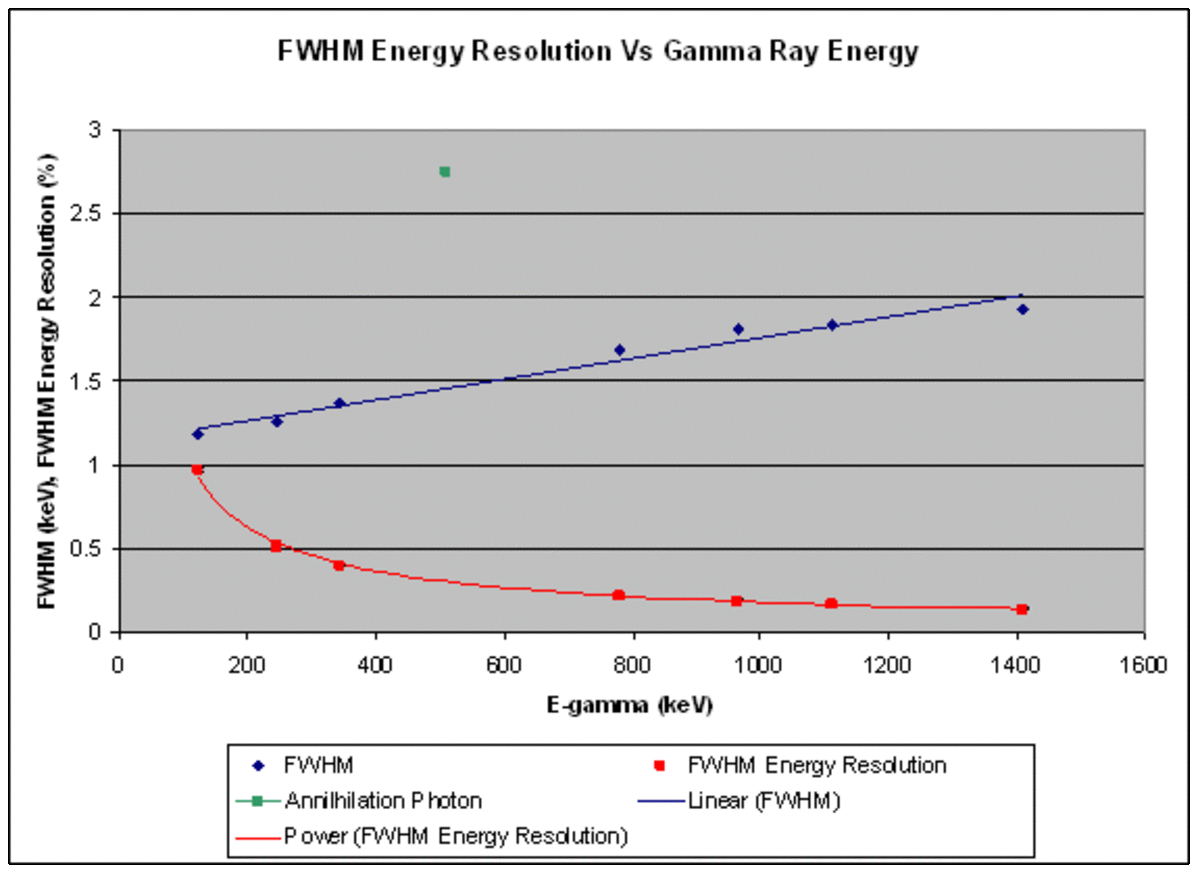Full width at half maximum (FWHM) and energy resolution for europium-152 peaks.

## Dead Time and Shaping Time

The dead time is the time for the detection system to reset after one event in order to receive another event. If radiation reaches the detector in this time then it will not be recorded as an event. A long shaping time for the amplifier will increase energy resolution, but with a high count rate there can be a pile-up of events leading to peak summing. Thus, the optimum shaping time is low for high count rates.

The graph below shows how with a constant shaping time, the dead time increases for high count rates. The count rate was increased by moving the 152Eu source closer to the detector; distances of 5, 7.5, 10 and 15 cm were used. The dead-time was determined by monitoring the MCA computer interface, and assessing the average dead time by eye. The large uncertainty is associated with the dead-time measurement being to 1 s.f. (as allowed by the interface).

## Absolute Total Efficiency

The absolute total efficiency (εt) of the detector is given by: εt = Ct ⁄ Nγ (×100%).

The quantity Ct is the total number of counts recorded per unit time, integrated over the whole spectrum. Nγ is the number of gamma rays emitted by the source per unit time. For a 152Eu source, the total number of counts recorded in 302 seconds of data collection was: 217,343±466, with a source-detector distance of 15 cm. The background count was 25,763±161. The total number of counts is therefore 191,580±493, with this error arising from a simple propagation of errors calculation √(a2+b2). Thus, per unit time, Ct = 634±2.

The number of gamma rays emitted per unit time is: Nγ = DS.Iγ(Eγ).

The quantity Iγ(Eγ) is the fractional number of gamma rays emitted per disintegration, which for 152Eu is 1.5. The quantity DS is the disintegration rate of the source (the activity). The original activity of the source was 370 kBq in 1987.

After 20.7 years and a half-life of 13.51 years, the activity at the time of this study is: DS = 370000 ⁄ 2(20.7 ⁄ 13.51) = 127.9±0.3 kBq.

Therefore, Nγ = 191900±500, and the absolute total efficiency is εt = 0.330±0.001%.

## Intrinsic Total Efficiency

The intrinsic total efficiency (εi) of the detector is given by: εi = Ct ⁄ Nγ'.

The quantity Nγ' is the total number of gamma rays incident on the detector, and is equal to: Nγ'= (Ω/4π)Nγ.

The quantity Ω is the solid angle subtended by the detector crystal at the point source, equalling: Ω = 2π.{1-[d ⁄ √(d2+a2)]}, where d is the distance from the detector to the source and a is the radius of the detector window.

For this study: Ω = 2π.{1-[150 ⁄ √(1502+302)]} = 0.039π.

Therefore Nγ' = 1871±5, and the intrinsic total efficiency, εi = 33.9±0.1%.

## Intrinsic Photopeak Efficiency

The intrinsic photopeak efficiency (εp) of the detector is: εp = Cp ⁄ Nγ'' (×100%).

The quantity Cp is the number of counts per unit time within a peak of energy Eγ. The quantity Nγ'' = Nγ' but with Iγ(Eγ) being the fractional number of gamma rays emitted with energy Eγ. Data and Iγ(Eγ) values are listed below for eight of the more prominent peaks in 152Eu.

E-gamma (keV)CountsCounts/secI-gammaN-gamma''Efficiency (%)

45.26

16178.14

53.57

0.169

210.8

25.41

121.78

33245.07

110.083

0.2837

354

31.1

244.7

5734.07

18.987

0.0753

93.9

20.22

344.27

14999.13

49.666

0.2657

331.4

14.99

778.9

3511.96

11.629

0.1297

161.8

7.19

964.1

3440.08

11.391

0.1463

182.5

6.24

1112.1

2691.12

8.911

0.1354

168.9

5.28

1408

3379.98

11.192

0.2085

260.1

4.3

The graph below shows the relationship between gamma ray energy and intrinsic photopeak efficiency. It is clear that efficiency decreases for higher energy gamma rays. This is due to the increased probability of rays not stopping within the detector. The efficiency also decreases at the lowest energies due to an increased probability of rays not reaching the depletion region of the detector.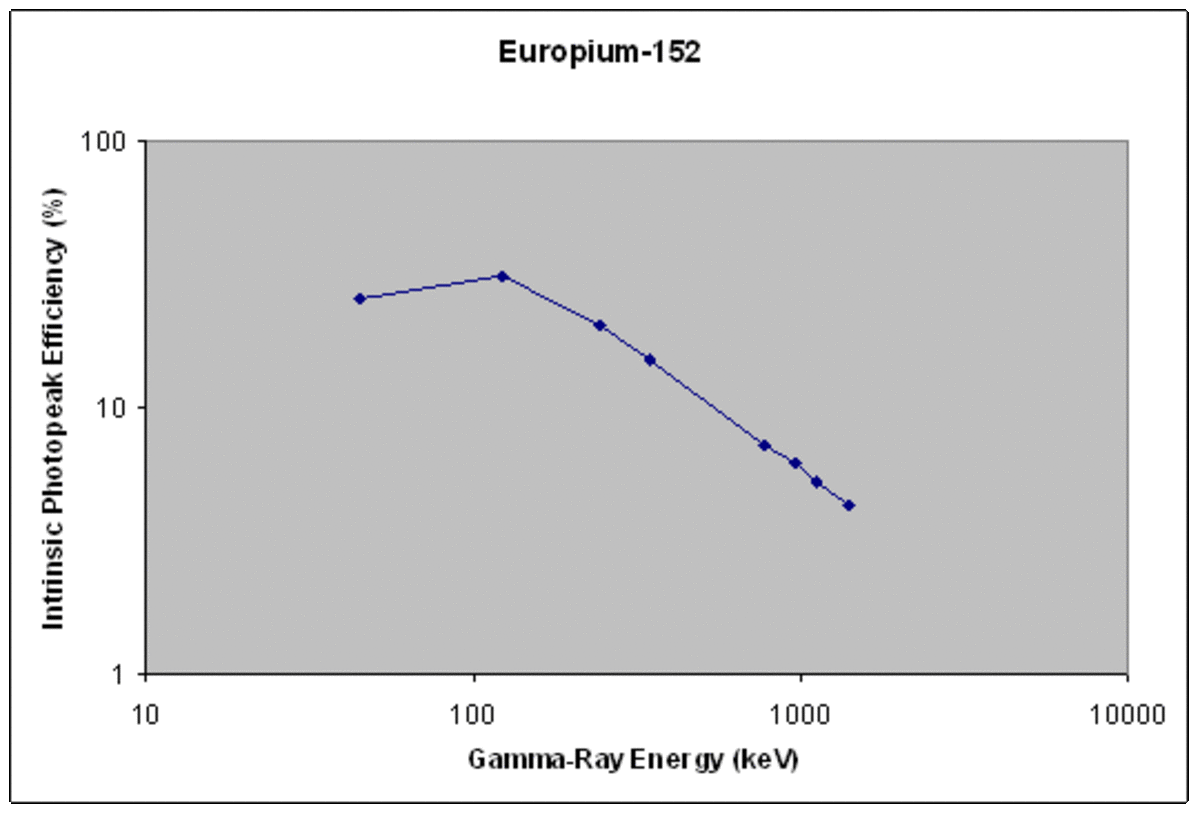A typical efficiency curve (intrinsic photopeak efficiency) for a europium-152 source.

## Summary

Gamma ray spectroscopy provides a fascinating look into the world beneath the scrutiny of our senses. To study gamma ray spectroscopy is to learn all the tools that are needed to become a proficient scientist. One must combine a grasp of statistics with a theoretical understanding of physical laws, and an experimental familiarity with scientific equipment. Nuclear physics discoveries utilizing gamma ray detectors continue to be made, and this trend looks set to continue well into the future.

Thomas Swan (author) from New Zealand on May 04, 2013:

Hi Rocket City Writer, this wasn't really research; it was some work I had to do in the lab to demonstrate an understanding of gamma-ray spectroscopy so that I would be able to do my research. Ultimately, I used some of these skills later though. My research was looking at nuclear isomers (which emit gamma rays).

I don't think I've used the same detectors as you have. I used silicon particle detectors during my research year to detect protons from a (d,p) reaction. It was nice to get an experience of more than one type of detector.

It's good to find a fellow physicist here. I'll check out some of your hubs. Thanks for commenting.

RocketCityWriter from Alabama on May 03, 2013:

Wow, so I stumbled onto one of your other hubs about quitting physics and then this one caught my eye.